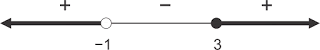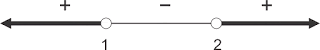# Solving Rational Inequalities (Algebra)

usa.m4thguru.info ~ Solving Rational Inequalities (Algebra). material is short and easy to understand with examples of rational inequality and fractions with easy-to-understand and clear discussion

Discussion of Materials and Questions of Rational Inequality and Fractions

Rational inequality

Rational inequality is a form of inequality that contains a rational function, which is a function that can be expressed in the form $$\mathrm{\frac{f(x)}{g(x)}}$$ dengan syarat g(x) ≠ 0.

Common forms of rational inequality:

$$\mathrm{\frac{f(x)}{g(x)}}$$ > 0  atau  $$\mathrm{\frac{f(x)}{g(x)}}$$ ≥ 0  ; g(x) ≠ 0

$$\mathrm{\frac{f(x)}{g(x)}}$$ < 0  atau  $$\mathrm{\frac{f(x)}{g(x)}}$$ ≤ 0  ; g(x) ≠ 0.

Here are some things that are not allowed in simplifying the form of rational inequality because it will change the domain of the function:

1. Cross product  $$\mathrm{\frac{f(x)}{g(x)}> c\;\;{\color{Red} \not\equiv}\;\; f(x)>c\,.\,g(x)}$$

2. Delete the same function or factor on the counter and denominator $$\mathrm{\frac{f(x)\,.\,g(x)}{g(x)}> c\;\;{\color{Red} \not\equiv}\;\; f(x)>c}$$

The set of resolutions of a rational inequality can be determined by the following steps:

State in general form.

Specify the zero maker on the counter and denominator.

Write a zero maker on the number line and specify a mark for each interval on the number line.

Determine the settlement area. For inequality ">" or "≥" the settlement area is at the interval marked positive and for the inequality "<" or "≤" the settlement area is at the interval marked negaitf.

Paying attention to the condition that the denominator is not equal to zero, write a set of completions that is the interval that contains the settlement area.

Example 1

The final answer of $$\mathrm{\frac{x-3}{x+1}}$$ ≥ 0

Zero maker:

Zeros of the numerator

x - 3 = 0 ⇒ x = 3

Zeros of the denominator

x + 1 = 0 ⇒ x = −1

Requirement :

x + 1 ≠ 0 ⇒ x ≠ −1

For the interval x <−1, take x = −2:

$$\mathrm{\frac{-2-3}{-2+1}}$$ = 5 (+)

For the interval −1 <x ≤ 3, take x = 0:

$$\mathrm{\frac{0-3}{0+1}}$$ = −3 (−)

For the interval x> 3, take x = 4:

$$\mathrm{\frac{4-3}{4+1}}$$ = $$\frac{1}{5}$$ (+)Due to the inequality marked "≥", the settlement area is at the interval marked (+).

The final answer in interval notation should be = {x <−1 or x ≥ 3}

Example 2

the final answer from $$\mathrm{\frac{2x-1}{4-x}}$$ > 0

Zero maker:

Zeros of the numerator

2x - 1 = 0 ⇒ x =  $$\frac{1}{2}$$

Zeros of the denominator

4 - x = 0 ⇒ x = 4

Requirement :

4 - x ≠ 0 ⇒ x ≠ 4Due to the inequality marked ">", the settlement area is at the interval marked (+).

The final answer in interval notation should be = {$$\frac{1}{2}$$ < x < 4}

Example 3

The final answer  from $$\mathrm{\frac{x^{2}-2x+1}{x+2}< 0}$$

$$\mathrm{\frac{(x-1)(x-1)}{x+2}<0}$$

Zero maker:

Zeros of the numerator

(x - 1) (x - 1) = 0 ⇒ x = 1

Zeros of the denominator

x + 2 = 0 ⇒ x = −2

Requirement :

x + 2 ≠ 0 ⇒ x ≠ −2Due to the inequality marked "<", the settlement area is at the interval marked (-).

The final answer in interval notation should be= {x <−2}

Example 4

The final answer from$$\mathrm{\frac{x-5}{x^{2}+6x+9}\leq 0}$$

$$\mathrm{\frac{x-5}{(x+3)(x+3)}\leq 0}$$

Zero maker:

Zeros of the numerator

x - 5 = 0 ⇒ x = 5

Zeros of the denominator

(x + 3) (x + 3) = 0 ⇒ x = −3

Requirement :

(x + 3) (x + 3) ≠ 0 ⇒ x ≠ −3Due to the inequality marked "≤", the settlement area is at the interval marked (-).

∴ The final answer in interval notation should be = {x <−3 or −3 <x ≤ 5} or

The final answer in interval notation should be = {x ≤ 5 and x ≠ −3}

From the above examples we can conclude as follows:

The zero maker on the denominator is always represented by an empty circle whatever the sign of inequality.

Signs for each interval are always alternating positive and negative if the inequality contains different linear factors (examples 1 and 2).

The marks for each interval become non-intermittent if the inequality contains the same linear factors (examples 3 and 4).

Rational inequality that contains definite functions

In the quadratic function material we recognize the existence of a function that is always positive value for each x real number (positive definite) and a function that is always negative value for each x real number (negative definition).

The positive definite function in a rational inequality can be ignored without having to reverse the sign of inequality.

Example 5

The final answer from$$\mathrm{\frac{x-4}{x^{3}+x}\leq 0}$$

$$\mathrm{\frac{x-4}{x(x^{2}+1)}\leq 0}$$

x2 + 1 is a positive definite function, can be proved by the positive definite terms namely: a> 0 and D <0.

Thus, x2 + 1 can be ignored without having to reverse the sign of inequality, so that the above inequality equals:

$$\mathrm{\frac{x-4}{x}\leq 0}$$

Zero maker:

Zeros of the numerator

x - 4 = 0 ⇒ x = 4

Zeros of the denominator

x = 0

Requirement :

x ≠ 0Due to the inequality marked "≤", the settlement area is at the interval marked (-).

∴The final answer in interval notation should be = {0 <x ≤ 4}

The negative definite function in a rational inequality can be ignored provided that the inequality sign must be changed or reversed.

Example 6

the final answer from $$\mathrm{\frac{-x^{2}+x-2}{x^{2}-4x+3}\leq 0}$$

$$\mathrm{\frac{-x^{2}+x-2}{(x-1)(x-3)}\leq 0}$$

−x2 + x - 2 is a negative definite function

, it can be proven by the negative definite conditions, namely: a <0 and D <0.

So, −x2 + x - 2 can be ignored provided that the sign of the inequality must be changed or reversed, so that the above inequality is equivalent to:

$$\mathrm{\frac{1}{(x-1)(x-3)}\geq 0}$$

Zero maker:

Zeros of the denominator

(x - 1) (x - 3) = 0 ⇒ x = 1 or x = 3

Terms:

(x - 1) (x - 3) ≠ 0 ⇒ x ≠ 1 or x ≠ 3Since the inequality is marked with "≥", the solution region is at the interval marked (+).

∴ The final answer in interval notation should be = {x <1 or x> 3}

Rational Inequalities Exercises

Exercise 1

Find the final answer of  $$\mathrm{\frac{2x-1}{x+2}\geq 1}$$

⇔ $$\mathrm{\frac{2x-1}{x+2}}$$ − 1 ≥ 0
⇔ $$\mathrm{\frac{2x-1}{x+2}-\frac{x+2}{x+2}}$$ ≥ 0
⇔ $$\mathrm{\frac{2x-1-x-2}{x+2}}$$ ≥ 0
⇔ $$\mathrm{\frac{x-3}{x+2}}$$ ≥ 0

Zero maker:

Zeros of the numerator

x - 3 = 0 ⇒ x = 3

Zeros of the denominator

x + 2 = 0 ⇒ x = −2

Terms:

x + 2 ≠ 0 ⇒ x ≠ −2Since the inequality is marked with "≥", the solution region is at the interval marked (+).

∴ The final answer in interval notation should be = {x <−2 or x ≥ 3}

Exercise 2

Find the final answer of $$\mathrm{2>\frac{5x}{x-4}}$$

The above inequality can be written as:

$$\mathrm{\frac{5x}{x-4}}$$ < 2

⇔ $$\mathrm{\frac{5x}{x-4}}$$ − 2 < 0
⇔ $$\mathrm{\frac{5x}{x-4}-\frac{2(x-4)}{x-4}}$$ < 0
⇔ $$\mathrm{\frac{5x-2x+8}{x-4}}$$ < 0
⇔ $$\mathrm{\frac{3x+8}{x-4}}$$ < 0

Zero maker:

Zeros of the numerator

3x + 8 = 0  ⇒ x = $$-\frac{8}{3}$$

Zeros of the denominator

x - 4 = 0 ⇒ x = 4

Terms:

x - 4 ≠ 0 ⇒ x ≠ 4

Since the inequality is marked with "<", the solution region is at the interval marked (-).

∴ The final answer in interval notation should be = {$$-\frac{8}{3}$$ < x < 4}

Exercise 3

Find the final answer of $$\mathrm{\frac{1-3x}{2x-2}\leq \frac{1}{2}}$$

⇔ $$\mathrm{\frac{1-3x}{2x-2}-\frac{1}{2}}$$ ≤ 0
⇔ $$\mathrm{\frac{1-3x}{2(x-1)}-\frac{1(x-1)}{2(x-1)}}$$ ≤ 0
⇔ $$\mathrm{\frac{1-3x-x+1}{2(x-1)}}$$ ≤ 0
⇔ $$\mathrm{\frac{2-4x}{2(x-1)}}$$ ≤ 0

Zero maker:

Zeros of the numerator

2 - 4x = 0 ⇒ x =  $$\frac{1}{2}$$

Zeros of the denominator

x - 1 = 0 ⇒ x = 1

Terms:

x - 1 ≠ 0 ⇒ x ≠ 1Since the inequality is marked with "≤", the solution region is at the interval marked (-).

∴ The final answer in interval notation should be = {x ≤ $$\frac{1}{2}$$ atau x > 1}

Exercise 4

Find the final answer of $$\mathrm{\frac{x^{2}+3x}{x+1}\geq 3}$$

⇔ $$\mathrm{\frac{x^{2}+3x}{x+1}}$$ − 3 ≥ 0
⇔ $$\mathrm{\frac{x^{2}+3x}{x+1}}$$ − $$\mathrm{\frac{3(x+1)}{x+1}}$$ ≥ 0
⇔ $$\mathrm{\frac{x^{2}+3x-3x-3}{x+1}}$$ ≥ 0
⇔ $$\mathrm{\frac{x^{2}-3}{x+1}}$$ ≥ 0
⇔ $$\mathrm{\frac{(x+\sqrt{3})(x-\sqrt{3})}{x+1}}$$ ≥ 0

Zero maker:

Zeros of the numerator

(x + √3) (x - √3) = 0 ⇒ x = √3 or x = −√3

Zeros of the denominator

x + 1 = 0 ⇒ x = −1

Terms:

x + 1 ≠ 0 ⇒ x ≠ −1Since the inequality is marked with "≥", the solution region is at the interval marked (+).

∴ The final answer in interval notation should be = {−√3 ≤ x <−1 or x ≥ √3}

Exercise 5

Find the Final answer of  $$\mathrm{\frac{x+1}{x-2}\geq \frac{1}{x-1}}$$

⇔ $$\mathrm{\frac{x+1}{x-2}-\frac{1}{x-1}}$$ ≥ 0
⇔ $$\mathrm{\frac{(x+1)(x-1)-(x-2)}{(x-2)(x-1)}}$$ ≥ 0
⇔ $$\mathrm{\frac{x^{2}-1-x+2}{(x-2)(x-1)}}$$ ≥ 0
⇔ $$\mathrm{\frac{x^{2}-x+1}{(x-2)(x-1)}}$$ ≥ 0

x2 − x + 1 is a positive definite function, so it can be ignored without changing or reversing the sign of the inequality.

So the above inequality is equivalent to

$$\mathrm{\frac{1}{(x-2)(x-1)}}$$ ≥ 0

Zero maker:

Zeros of the denominator

(x - 2) (x - 1) = 0 ⇒ x = 2 or x = 1

Terms:

(x - 2) (x - 1) ≠ 0 ⇒ x ≠ 2 or x ≠ 1Since the inequality is marked with "≥", the solution region is at the interval marked (+).

∴The final answer in interval notation should be = {x <1 or x> 2}

Exercise 6

Find the final answer of$$\mathrm{\frac{4}{-x^{2}-4}\geq \frac{1}{x-1}}$$

⇔ $$\mathrm{\frac{4}{-x^{2}-4}-\frac{1}{x-1}}$$ ≥ 0
⇔ $$\mathrm{\frac{4(x-1)-(-x^{2}-4)}{(-x^{2}-4)(x-1)}}$$ ≥ 0
⇔ $$\mathrm{\frac{4x-4+x^{2}+4}{(-x^{2}-4)(x-1)}}$$ ≥ 0
⇔ $$\mathrm{\frac{x^{2}+4x}{(-x^{2}-4)(x-1)}}$$ ≥ 0
⇔ $$\mathrm{\frac{x(x+4)}{(-x^{2}-4)(x-1)}}$$ ≥ 0

−x2 − 4  is a negative definite function, so it can be ignored provided the inequality sign is changed or reversed.

So the above inequality is equivalent to

$$\mathrm{\frac{x(x+4)}{x-1}}$$ ≤ 0

Zeros of the numerator

x (x + 4) = 0 ⇒ x = 0 or x = −4

Zeros of the denominator

x - 1 = 0 ⇒ x = 1

Terms:

x - 1 ≠ 0 ⇒ x ≠ 1Since the inequality is marked with "≤", the solution area is at the interval marked (-).

∴ The final answer in interval notation should be = {x ≤ −4 or 0 ≤ x <1}

such is the Solving Rational Inequalities (Algebra). may be useful

LihatTutupKomentar